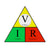0

Ohm’s Law For Vaping

December 15, 2017 3 min readOhm’s Law For Vaping

When it comes to Ohm’s law there are three main elements that you have to know and be aware of and they are

• • V = Voltage (your battery voltage)
• • I = Current (the amperage drawn by your coil)
• • R = Resistance (the resistance, in ohms, of your coil)

This is commonly viewed as a triangle which can be described as V (Voltage) over I (Current) multiplied by R (Resistance). So what does that mean and how do you go about using them to build your coils for a safe vaping experience. There are a few different ways you can go about this and they are the following

• • Calculate the current
• • Calculate the wattage (power)
• • Calculate the resistance

Calculate the current

To calculate the amount of current that is going to be drawn through the resistance which is your coil you need to use the following equation.

I (current) = V (Voltage) / R (Resistance)

So for example if you are using a freshly charged battery with a 4.2V and your coil is 0.5Ω you would simply do the following

I = 4.2V / 0.5Ω

I= 8.4A

This therefore means that the total max current being drawn is 8.4 amps. If your battery has an amp limit of 10 amps, then you are below the capacity of that battery. Don’t forget that using a dual mechanical mod in series configuration will double your amp draw per battery, and you will have to build coils with twice as high resistance to be safe. Also note that as the battery depletes, the current will also tail off. For example, when the battery reaches 3.7 volts with the same load, current will drop to 7.4 amps (3.7 volts / 0.5 ohms)

Calculate the wattage (power)

Calculating the wattage allows you to see the power generated by your coil. In order to do this, you multiply the I (current) by the V (voltage). The equation is as follows

P = V(voltage) x I (current)

So again using the theory of a freshly charged battery with 4.2V

P = 4.2V x 8.4A

P = 35.3W

So if you run a coil at 0.5Ω with a fully charged battery at 4.2V it will pull a max of 8.4 amps which will deliver 35.3 watts. If the resistance of your coil increases, both the current and wattage will drop.

Example

I = 4.2V / 0.9Ω

I = 4.6A

Take this and the use the formula we just explained and you will get the following

P = 4.2V x 4.6A

P = 19.32W

Calculate the resistance

Ok so let’s say that you have a battery that has a 10amp current limit and you want to work out the safest lowest resistance of a coil you can use without exceeding the batteries capabilities or the CDR (Continuous Discharge Rating) of the battery, you will need to use the following equation

R(resistance) = V(voltage) / I(current)

Always allow yourself some safety room, for example if you know the battery is a 10amp battery then stay on the side of safety and give yourself some room by dropping it by 1amp in your equation. The equation is as follows

R = 4.2 / 9A

R= 0.47Ω

You have just calculated that lowest safe limit with a 10amp battery is a coil with 0.47Ω anything lower than this resistance you risk exceeding the current limit of the battery. However, if your battery is a 20amp battery then you could go as low as 0.22Ω, see equation below

R = 4.2 / 19A

R = 0.22Ω

All this is great stuff to know to ensure you stay within the safety limits of your batteries capacity when building your coils. There are also a wide range of useful apps and sites that can assist you with Ohms law. One in particular is Steam Engine which also has information such as coil ramp up time, coil gauges etc. Make sure you equip yourself with battery safety knowledge and always test your coils on a ohm reader prior to installing them.